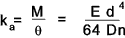# Torsion constant of a bent spring

How do I find torsion constant (Kt) of a spring which is bent, as shown in the image below

#### Attachments

•piyush1.jpg
9.1 KB · Views: 611

jack action
Gold Member
$$K_t= \frac{T}{\theta}= \frac{Fr}{\frac{x}{r}}= \frac{F}{x}r^2= Kr^2$$

Where $K$ is the linear stiffness of the spring and $r$ is the distance between the spring and the center of rotation.

Hello,
May be I wasn't clear in previous post. The spring is being rotated along its axis, while being kept in a curved position. In this case how will the torsion constant change ?

jack action
Gold Member

I'm going to let other chimes in, but I don't think it change anything for the torsional stiffness; Just like the bent doesn't change anything to linear stiffness (for example, the way I thought you were using the spring). The torsional stiffness is:and none of these variables should change if the spring is bent. The exception would be if the bent deformed the spring so much that it doesn't allow the spring to freely rotate. For example, if the bent is so pronounced that it looks like 2 springs side by side joint by a wire, you will probably end up with 2 independent springs with half the active coils of the initial spring (i.e. twice the initial spring torsional stiffness).

However, because there is an initial deformation, the torsional stress limit that it can withstand will be lower.